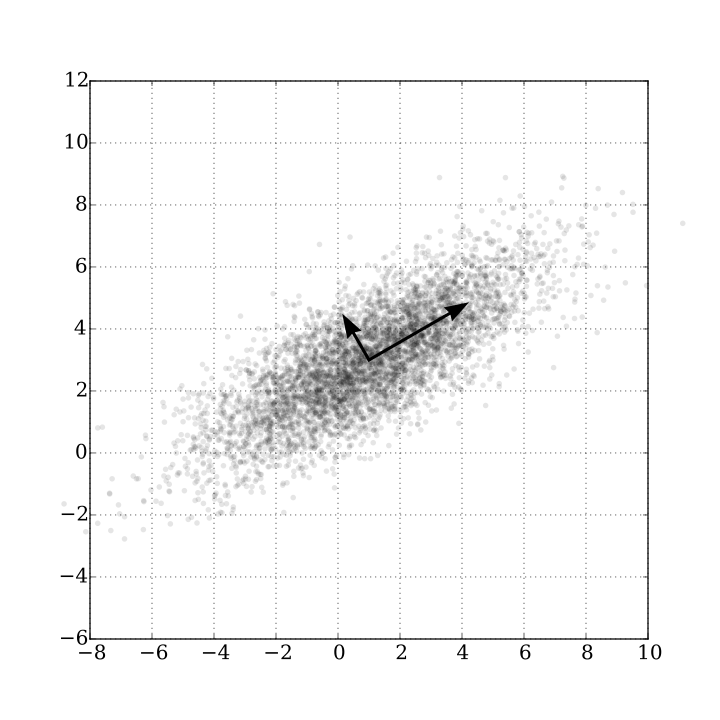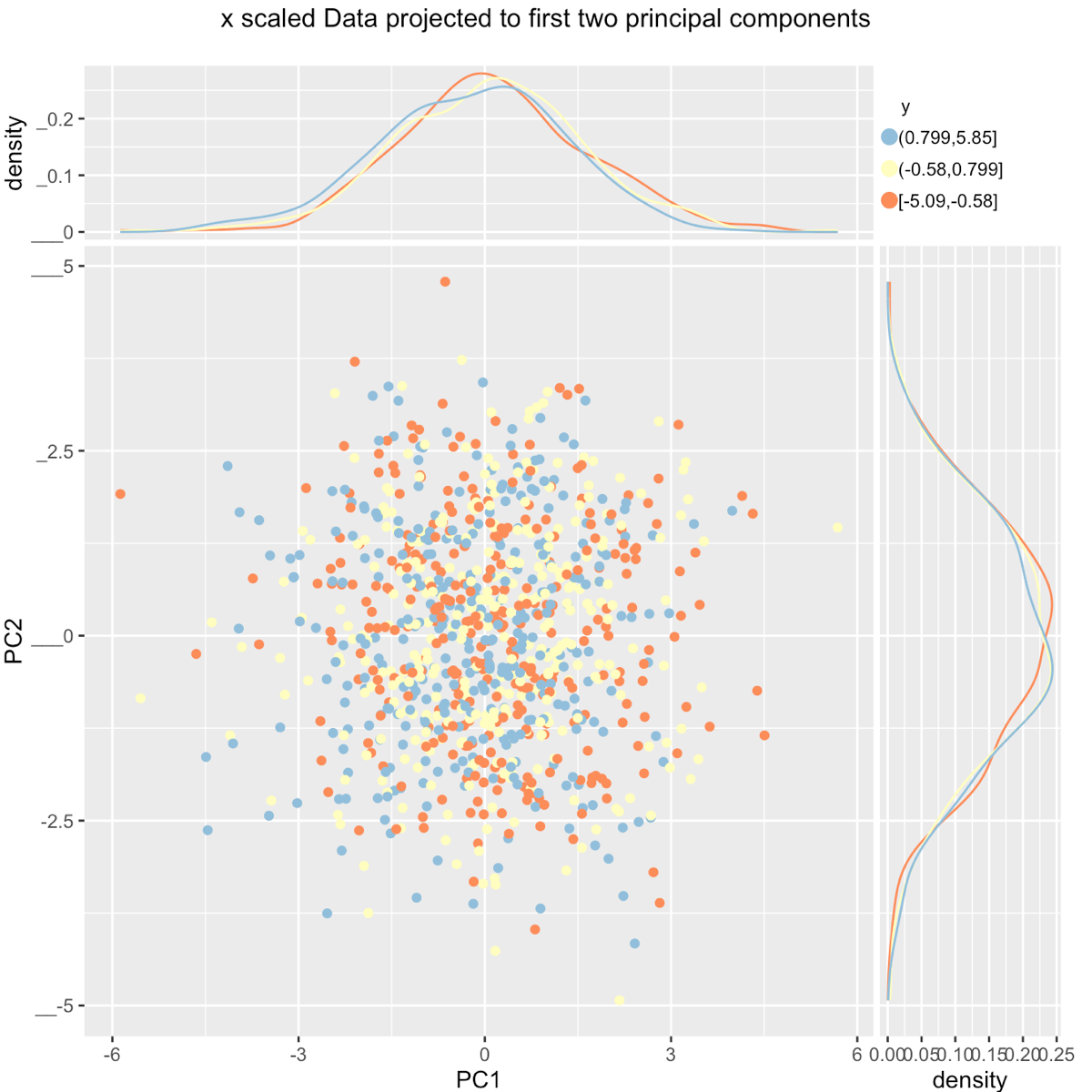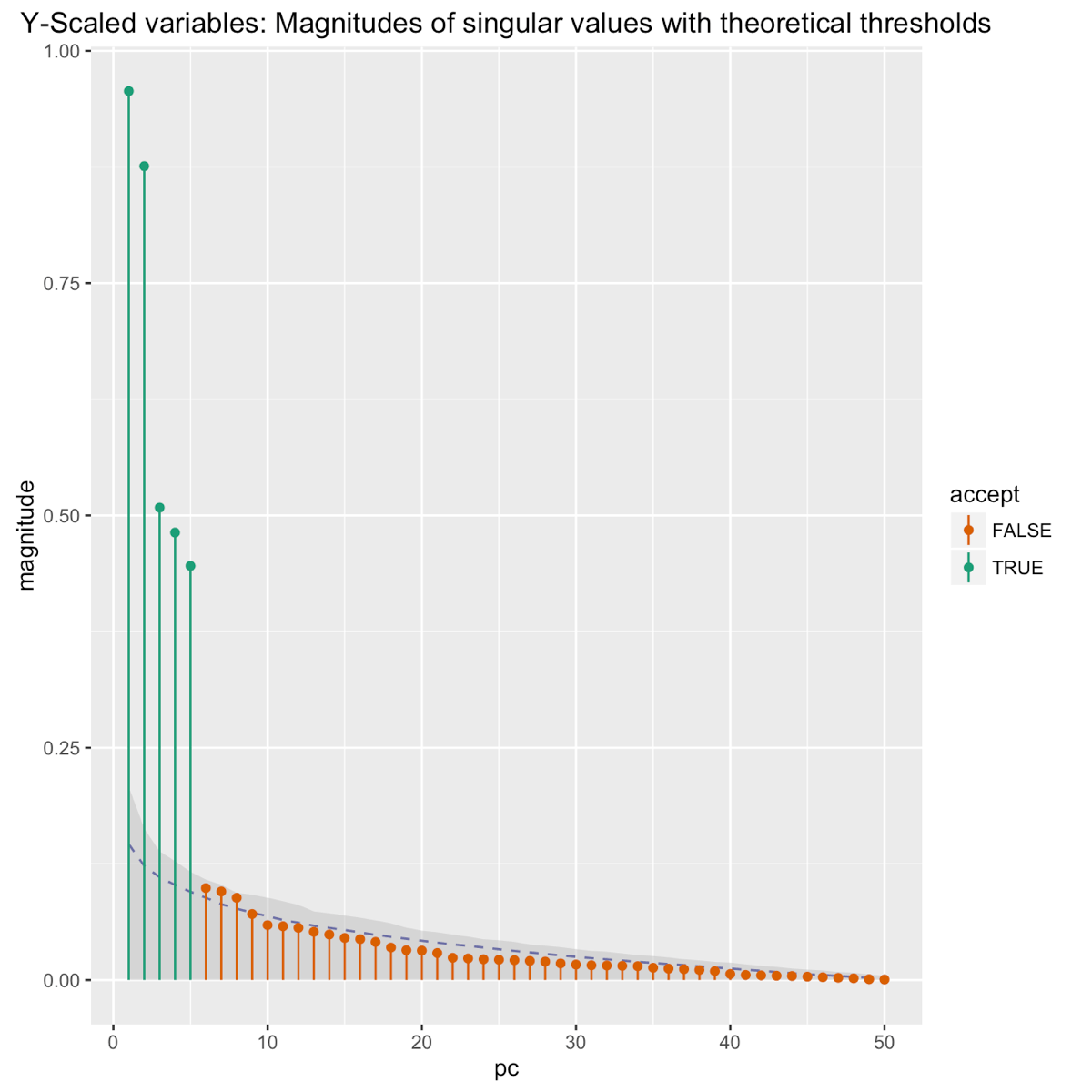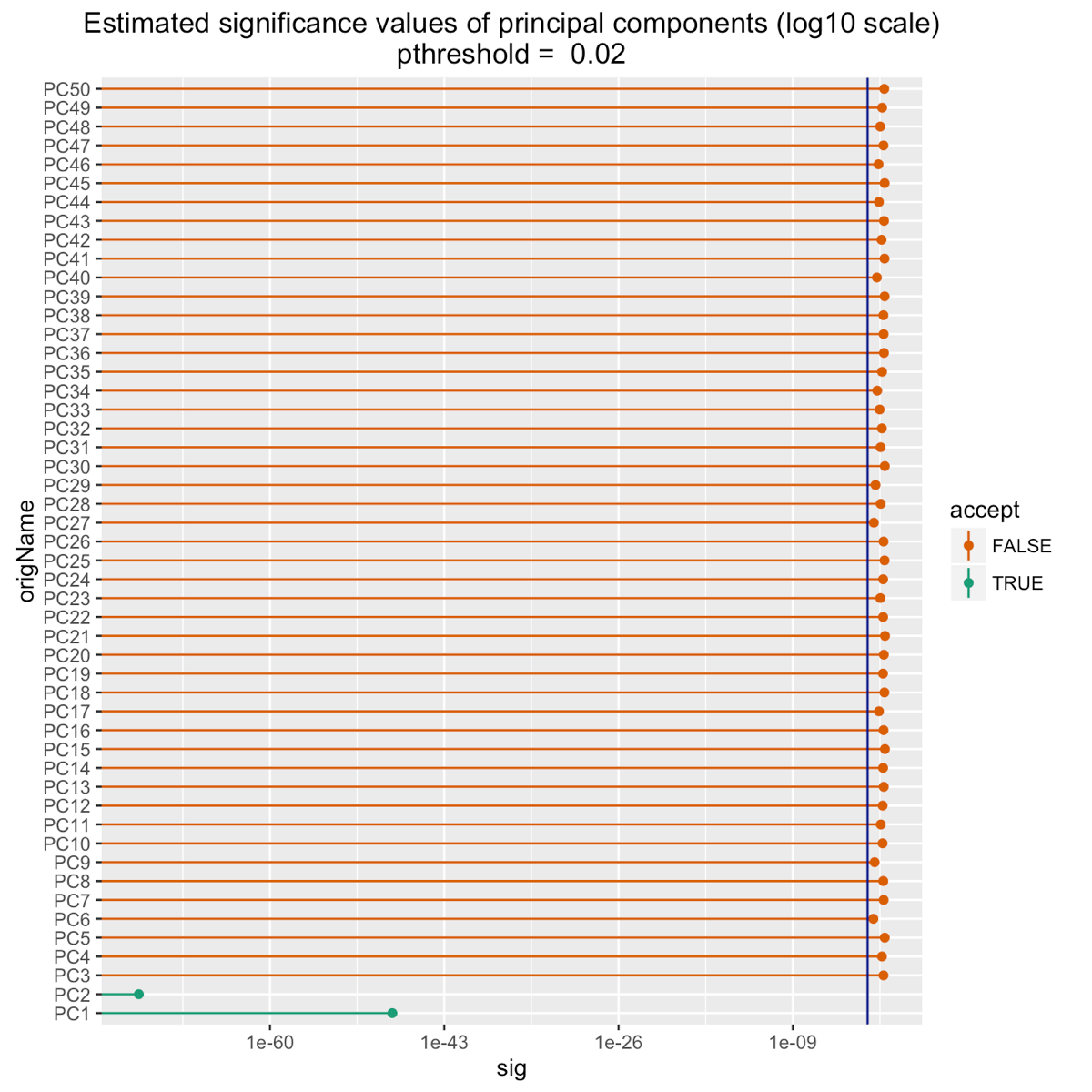# Short form:

Win-Vector LLC’s Dr. Nina Zumel has a three part series on Principal Components Regression that we think is well worth your time.

• Part 1: the proper preparation of data (including scaling) and use of principal components analysis (particularly for supervised learning or regression).
• Part 2: the introduction of y-aware scaling to direct the principal components analysis to preserve variation correlated with the outcome we are trying to predict.
• Part 3: how to pick the number of components to retain for analysis.

# Part 1 Principal Components Regression/Analysis: The Standard Methods

You can read her first article part 1 here. Principal Components Regression (PCR) is the use of Principal Components Analysis (PCA) as a dimension reduction step prior to linear regression. It is one of the best known dimensionality reduction techniques and a staple procedure in many scientific fields. PCA is used because:

• It can find important latent structure and relations.
• It can reduce over fit.
• It can ease the curse of dimensionality.
• It is used in a ritualistic manner in many scientific disciplines. In some fields it is considered ignorant and uncouth to regress using original variables.

We often find ourselves having to often remind readers that this last reason is not actually positive. The standard derivation of PCA involves trotting out the math and showing the determination of eigenvector directions. It yields visually attractive diagrams such as the following.Wikipedia: PCA

And this leads to a deficiency in much of the teaching of the method: glossing over the operational consequences and outcomes of applying the method. The mathematics is important to the extent it allows you to reason about the appropriateness of the method, the consequences of the transform, and the pitfalls of the technique. The mathematics is also critical to the correct implementation, but that is what one hopes is already supplied in a reliable analysis platform (such as R). Dr. Zumel uses the expressive and graphical power of R to work through the use of Principal Components Regression in an operational series of examples. She works through how Principal Components Regression is typically mis-applied and continues on to how to correctly apply it. Taking the extra time to work through the all too common errors allows her to demonstrate and quantify the benefits of correct technique. Dr. Zumel will soon follow part 1 later with a shorter part 2 article demonstrating important "y-aware" techniques that squeeze much more modeling power out of your data in predictive analytic situations (which is what regression actually is). Some of the methods are already in the literature, but are still not used widely enough. We hope the demonstrated techniques and included references will give you a perspective to improve how you use or even teach Principal Components Regression. Please read on here.

# Part 2: Y-Aware Methods

In part 2 of her series on Principal Components Regression Dr. Nina Zumel illustrates so-called y-aware techniques. These often neglected methods use the fact that for predictive modeling problems we know the dependent variable, outcome or y, so we can use this during data preparation in addition to using it during modeling. Dr. Zumel shows the incorporation of y-aware preparation into Principal Components Analyses can capture more of the problem structure in fewer variables. Such methods include:

• Effects based variable pruning
• Significance based variable pruning
• Effects based variable scaling.

This recovers more domain structure and leads to better models. Using the foundation set in the first article Dr. Zumel quickly shows how to move from a traditional x-only analysis that fails to preserve a domain-specific relation of two variables to outcome to a y-aware analysis that preserves the relation. Or in other words how to move away from a middling result where different values of y (rendered as three colors) are hopelessly intermingled when plotted against the first two found latent variables as shown below.Dr. Zumel shows how to perform a decisive analysis where y is somewhat sortable by the each of the first two latent variable and the first two latent variables capture complementary effects, making them good mutual candidates for further modeling (as shown below).Click here (part 2 y-aware methods) for the discussion, examples, and references. Part 1 (x only methods) can be found here.

# Part 3: picking k and wrapping up

In her series on principal components analysis for regression in R Win-Vector LLC‘s Dr. Nina Zumel broke the demonstration down into the following pieces:

• Part 1: the proper preparation of data and use of principal components analysis (particularly for supervised learning or regression).
• Part 2: the introduction of y-aware scaling to direct the principal components analysis to preserve variation correlated with the outcome we are trying to predict.
• And now Part 3: how to pick the number of components to retain for analysis.

In the earlier parts Dr. Zumel demonstrates common poor practice versus best practice and quantifies the degree of available improvement. In part 3 she moves from the usual "pick the number of components by eyeballing it" non-advice and teaches decisive decision procedures. For picking the number of components to retain for analysis there are a number of standard techniques in the literature including:

• Pick 2, as that is all you can legibly graph.
• Pick enough to cover some fixed fraction of the variation (say 95%).
• (for variance scaled data only) Retain components with singular values at least 1.0.
• Look for a "knee in the curve" (the curve being the plot of the singular value magnitudes).
• Perform a statistical test to see which singular values are larger than we would expect from an appropriate null hypothesis or noise process.

Dr. Zumel shows that the last method (designing a formal statistical test) is particularly easy to encode as a permutation test in the y-aware setting (there is also an obvious similarly good bootstrap test). This is well-founded and pretty much state of the art. It is also a great example of why to use a scriptable analysis platform (such as R) as it is easy to wrap arbitrarily complex methods into functions and then directly perform empirical tests on these methods. The following "broken stick" type test yields the following graph which identifies five principal components as being significant:However, Dr. Zumel goes on to show that in a supervised learning or regression setting we can further exploit the structure of the problem and replace the traditional component magnitude tests with simple model fit significance pruning. The significance method in this case gets the stronger result of finding the two principal components that encode the known even and odd loadings of the example problem:In fact that is sort of her point: significance pruning either on the original variables or on the derived latent components is enough to give us the right answer. In general we get much better results when (in a supervised learning or regression situation) we use knowledge of the dependent variable (the "y" or outcome) and do all of the following:

• Model fit significance prune incoming variables.
• Convert incoming variables into consistent response units by y-aware scaling.
• Model fit significance prune resulting latent components.

The above will become much clearer and much more specific if you click here to read part 3.### jmount

Data Scientist and trainer at Win Vector LLC. One of the authors of Practical Data Science with R.